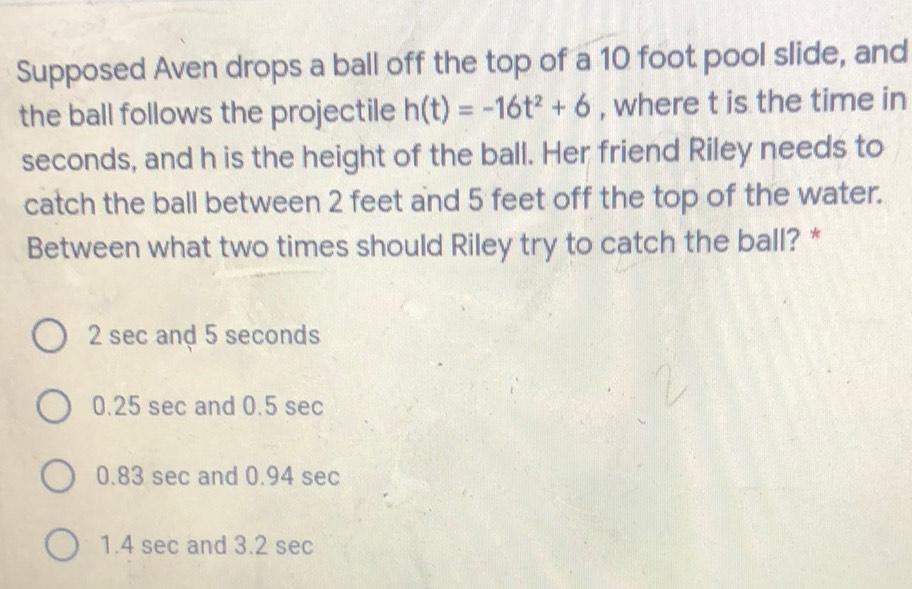Question:

# Supposed Aven drops a ball off the top of a 10 foot pool slide, and the ball follows the projectile h(t) = -16t? + 6, where t isSupposed Aven drops a ball off the top of a 10 foot pool slide, and the ball follows the projectile h(t) = -16t? + 6, where t is the time in seconds, and his the height of the ball. Her friend Riley needs to catch the ball between 2 feet and 5 feet off the top of the water. Between what two times should Riley try to catch the ball? * 2 sec and 5 seconds O 0.25 sec and 0.5 sec O 0.83 sec and 0.94 sec O 1.4 sec and 3.2 sec